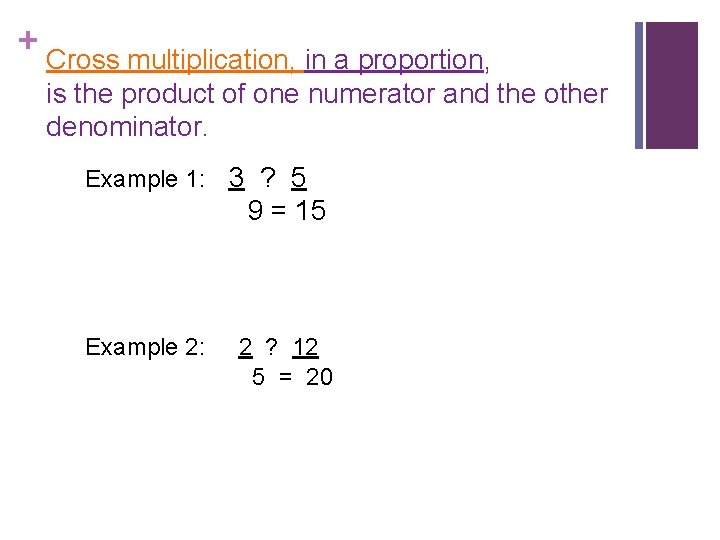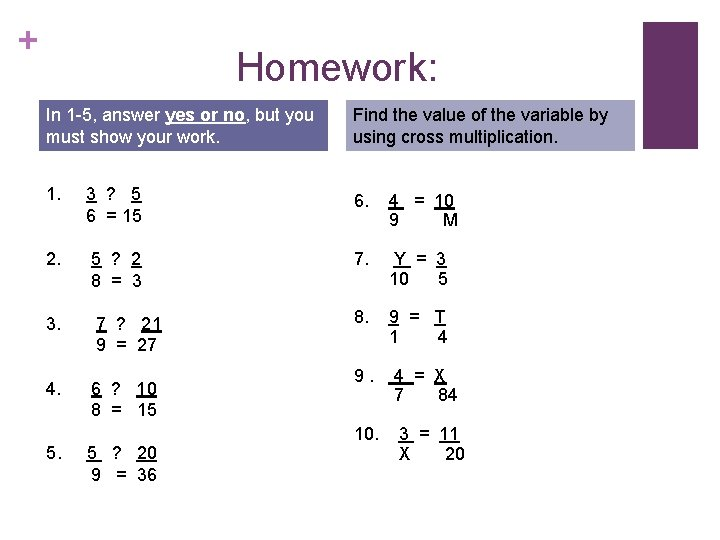# Finding Proportions using Cross Multiplication Objective We will

• Slides: 6Finding Proportions using Cross Multiplication + Objective: We will learn to use cross multiplication to solve a proportion. We will use cross multiplication to check whether two ratios form a proportion.+ Property of Cross Products: If two ratios form a proportion, the cross products are equal. When 2 ratios have equal cross products, they form a proportion. Example 1: n Decide whether each pair of ratios forms a proportion. You have to multiply the numerator with the denominator of the other fraction; and vice versa. 105= =105 n n n 3× 35 = 5 × 21 105 = 105 YES, they form a proportion. If the two products are equal, they form a proportion. When the cross products are not equal, the ratios do not form a proportion.+ Cross multiplication, in a proportion, is the product of one numerator and the other denominator. Example 1: 3 ? 5 9 = 15 Example 2: 2 ? 12 5 = 20+ How to Solve a Proportion: When solving a proportion you must cross multiply, then find the missing variable. n n n : 4 = 7 For example H 84 Steps: 7×H = 4× 84 7 H 1. Write the cross products. = 336 2. Multiply 7 H = 336 7 7 H = 48 3. To find the value of the variable, undo the multiplication by dividing to both sides. 4. Solve. 5. Write answer. (You have to think: The opposite of multiplication is division, so I have to divide the number that is with the variable and do the same for both sides of the problem. Then I cancel the opposites, and bring down what I have left. )+ Your Example: Solve the proportion. 2 = 3 4 N X = 1 6 3+ Homework: In 1 -5, answer yes or no, but you must show your work. Find the value of the variable by using cross multiplication. 1. 3 ? 5 6 = 15 6. 4 = 10 9 M 2. 5 ? 2 8 = 3 7. Y = 3 10 5 3. 7 ? 21 9 = 27 8. 9 = T 1 4 4. 6 ? 10 8 = 15 9. 4 = X 7 84 10. 3 = 11 X 20 5. 5 ? 20 9 = 36